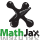Annualized total return calculator

 par value: 100 purchase price: sale price: coupon rate r : % current rate : coupons per year n : 1 2 3 4 6 12 purchase date: today sale date: today  sell maturity date: today  keep to maturity secondary rate s : % coupon reinvestment: none at the secondary rate in the bond calculate annualized total return: yield to maturity: coupon interest: final - price: total return: time of ownership t :

To compare bonds to growth investments, calculate the annualized total return.
Total return is the sum of all the interest earned by the bond, plus the difference between final (either par or the sale price) and price (the purchase price).
The coupon interest may be reinvested, either elsewhere at the secondary rate s, or in the bond at rate r.
The difference between final and price is an additional profit or loss which is incurred at maturity or sale.
Total the (reinvested) coupon interest I and final - price, and annualize the total as a percentage of price to get the annualized total return.

Yield to maturity is a special case of total return and must be calculated by successive approximation.
If the secondary rate is set to the yield to maturity, the latter will equal the total return compounded n times per year.

The calculations use a year length of 365.24 days, independent of the coupon dates and day count basis.

1.  coupon interest earned at rate r and reinvested at rate s:

% MathJax is loading . . . . \displaystyle \begin{alignat}{3} I &= a + ax + ax^2 + ax^3 + \cdots + ax^{m-1} \\[0.5em] &= \frac {a(1-x^m)} {1-x} &\textrm{if} \ \ x &≠ 1 \\[0.5em] &= am &\textrm{if} \ \ x &= 1 \end{alignat}where

\displaystyle \begin{align} & \textrm{coupon interest} \ \ a = par \cdot \frac {r} {n} \\[0.5em] & \textrm{secondary interest} \ \ x = 1 + \frac {s} {n} \\[0.5em] & m = nt \end{align}

2.  annualize I + (final - price) :

$$\displaystyle \textrm{annualized total return} = \sqrt [\Large \uproot{2} {t}] { \frac {I + (final - price)}{price} + 1 } - 1$$

3.  yield to maturity YTM by successive approximation:

\begin{align} & YTM = 0.1 \\[0.5em] & s = 0 \end{align}

$$\textrm{while} \ | YTM - s | > 0.000001$$

\begin{align} & s = YTM \\[0.5em] & x = 1 + \frac {s} {n} \\[0.5em] & I = \frac {a(1-x^m)} {1-x} \\[0.5em] & YTM = n \left( \sqrt [\Large \uproot{2} {nt}] { \frac {I + (final - price)}{price} + 1 } - 1 \right) \end{align}